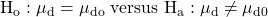IFT Notes for Level I CFA® Program
IFT Notes for Level I CFA® Program

# Part 2

## 3. Hypothesis Tests Concerning the Mean

### 3.1.     Tests Concerning a Single Mean

One of the decisions we need to make in hypothesis testing is deciding which test statistic and which corresponding probability distribution to use. We use the following table to make this decision:

 Sampling from Small sample size (n<30) Large sample size (n≥30) Normal distribution Variance known z z Variance unknown t t (or z) Non –normal distribution Variance known NA z Variance unknown NA t (or z)

In the hypothesis tests we saw so far, the population variance was known and our sample size was large (n≥30), hence we used the z-statistic and z-distribution to compute the critical value.

However, if we do not know the population variance and we have a small sample size, then we have to use the t-statistic and t-distribution to compute the critical values.

Example

Fund Alpha has been in existence for 20 months and has achieved a mean monthly return of 2% with a sample standard deviation of 5%. The expected monthly return for a fund of this nature is 1.60%.  Assuming monthly returns are normally distributed, are the actual results consistent with an underlying population mean monthly return of 1.60%?

Solution:

The null and alternative hypotheses for this example will be:

H0: µ = 1.60 versus Ha: µ ≠ 1.60Using this formula, we see that the value of the test statistic is 0.36.

The critical values at a 0.05 level of significance can be calculated from the t-distribution table. Since this is a two-tailed test, we should look at a 0.05/2 = 0.025 level of significance with df = n – 1 = 20 – 1 = 19. This gives us two values of -2.1 and +2.1.

Since our test statistic of 0.35 lies between -2.1 and +2.1, i.e., the acceptance region, we do not reject the null hypothesis.

### 3.2.     Tests Concerning Differences between Means

Instructor’s Note:

Focus on the basics of this topic, the probability of being tested on the details is low.

In this section, we will learn how to calculate the difference between the means of two independent and normally distributed populations. We can use two kinds of t-tests. In one case the population variances, although unknown, can be assumed to be equal. In the second case the population variances are assumed to be unknown and unequal.

Unknown But Equal Variance

When we assume that the two populations are normally distributed and that the unknown population variances are equal, the t-test based on independent random samples is given by:The termis known as the pooled estimator of the common variance. It is calculated by the following formula:The number of degrees of freedom is n1 + n2 – 2.

Unknown and Unequal Variance

When we can assume that the two populations are normally distributed and that the unknown population variances are unequal, an approximate t-test based on independent random samples is given by:In this formula, we use the tables of the t-distribution using the ‘modified’ degrees of freedom. The ‘modified’ degrees of freedom are calculated using the following formula:Example

You believe the mean return on NYSE stocks was different from the mean on NSE stocks last month. To test your hypothesis you collect the following data:

 Sample Size (n) Sample Mean (X̄) Sample Standard Deviation (s) NSE 20 2% 4 NYSE 40 3% 5

Determine whether to reject the null hypothesis at the 0.10 level of significance.

Solution:

The first step is to formulate the null and alternative hypotheses. Since we want to test whether the two means were equal or different, we define the hypotheses as:

H0: µ1 – µ2 = 0

Ha: µ1 – µ2 ≠ 0

Since the population standard deviation is unknown and we cannot assume that it is equal, we use the following formula to calculate the test statistic:Next, we calculate the modified degrees of freedom:For a 0.10 level of significance, we find the t-value for 0.10/2 = 0.05 using df = 48. The t-value is therefore ta/2= -1.677 and +1.677. Since our test statistic of -0.84 lies in the acceptance region, we fail to reject the null hypothesis.

### 3.3.     Tests concerning Mean Differences

Instructor’s Note:

Focus on the basics of this topic, the probability of being tested on the details is low.

In the previous section, in order to perform hypothesis tests on differences between means of two populations, we assumed that the samples were independent. What if the samples are not independent? For example, suppose you want to conduct tests on the mean monthly return on Toyota stock and mean monthly return on Honda stock. These two samples are believed to be dependent, as they are impacted by the same economic factors.

In such situations, we conduct a t-test that is based on data arranged in paired observations. Paired observations are observations that are dependent because they have something in common.

We will now discuss the process for conducting such a t-test. Suppose that we gather data regarding the mean monthly returns on stocks of Toyota and Honda for the last 20 months, as shown in the table below:

 Month Mean return of Toyota stock Mean monthly return of Honda stock Difference in mean monthly returns (di) 1 0.5% 0.4% 0.1% 2 0.7% 1.0% -0.3% 3 0.3% 0.7% -0.4% … … … … 20 0.9% 0.6% 0.3% Average 0.750% 0.600% 0.075%

Here is a simplified process for conducting the hypothesis test:

Step 1: Define the null and alternate hypotheses

We believe that the mean difference is not 0. Hence the null and alternate hypotheses are: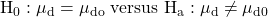µd stands for the population mean difference and µd0 stands for the hypothesized value for the population mean difference.

Step 2: Calculate the test-statistic

Determine the sample mean difference using: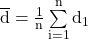For the data given, the sample mean difference is 0.075%.

Calculate the sample standard deviation. The process for calculating the sample standard deviation has been discussed in an earlier reading. The simplest method is to plug the numbers (0.1, -0.3, -0.4…0.3) into a financial calculator.  The entire data set has not been provided. We’ll take it as a given that the sample standard deviation is 0.150%.

Use this formula to calculate the standard error of the mean difference: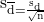For our data this is 0.150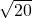= 0.03354.

We now have the required data to calculate the test statistic using a t-test. This is calculated using the following formula using n – 1 degrees of freedom: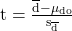For our data, the test statistic is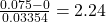Step 3: Determine the critical value based on the level of significance

We will use a 5% level of significance. Since this is a two-tailed test we have a probability of 2.5% (0.025) in each tail.  This critical value is determined from a t-table using a one-tailed probability of 0.025 and df = 20 – 1 = 19. This value is 2.093.

Step 4: Compare the test statistic with the critical value and make a decision

In our case, the test statistic (2.23) is greater than the critical value (2.093). Hence we will reject the null hypothesis.

Conclusion: The data seems to indicate that the mean difference is not 0.

The hypothesis test presented above is based on the belief that the population mean difference is not equal to 0. If  is the hypothesized value for the population mean difference, then we can formulate the following hypotheses:

1. If we believe the population mean difference is greater than 0:
2.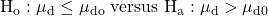1. If we believe the population mean difference is less than 0:
2.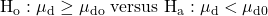1. If we believe the population mean difference is not 0:
2.